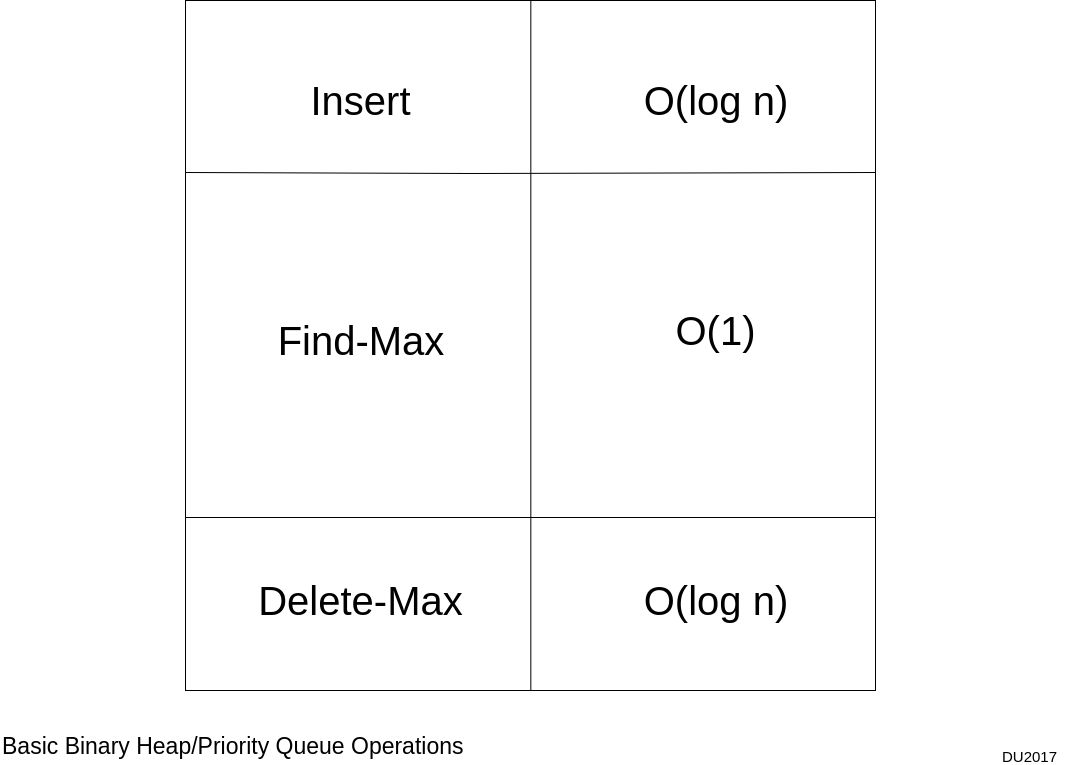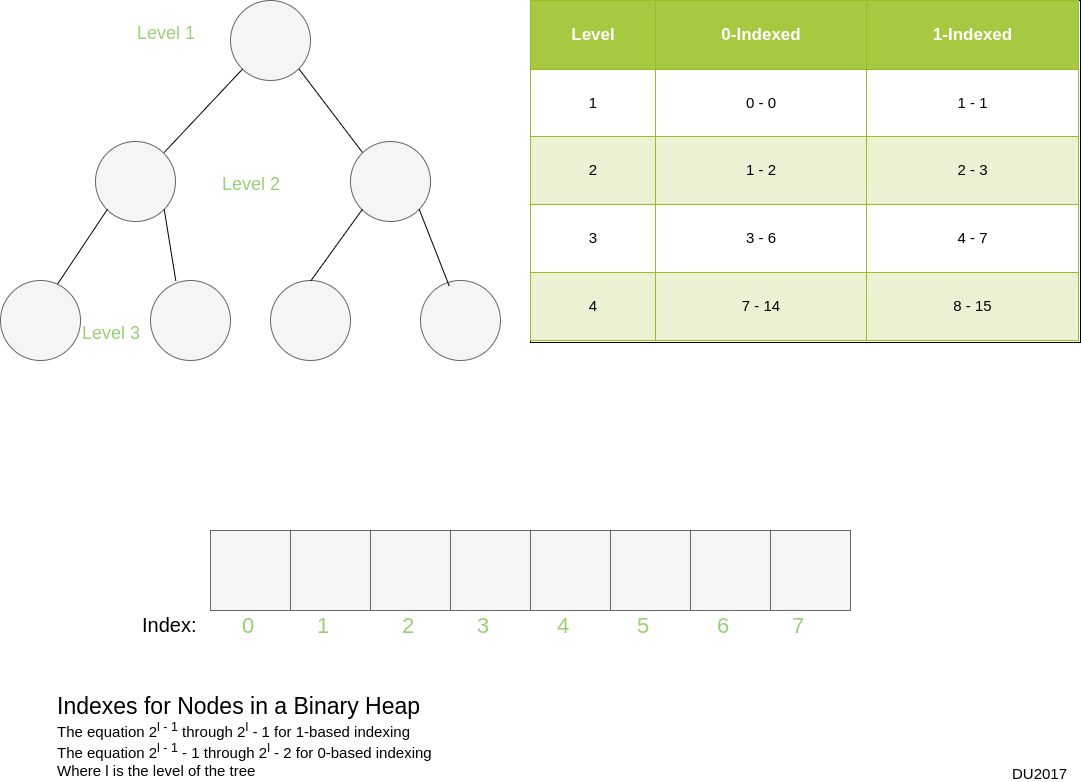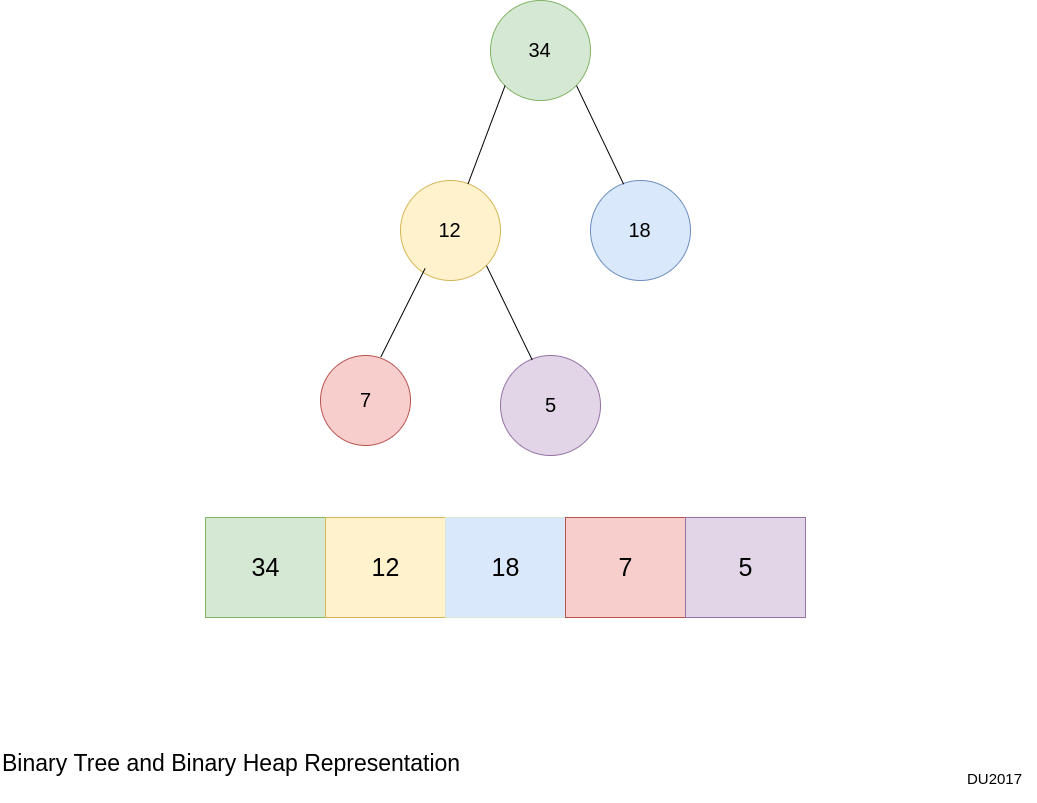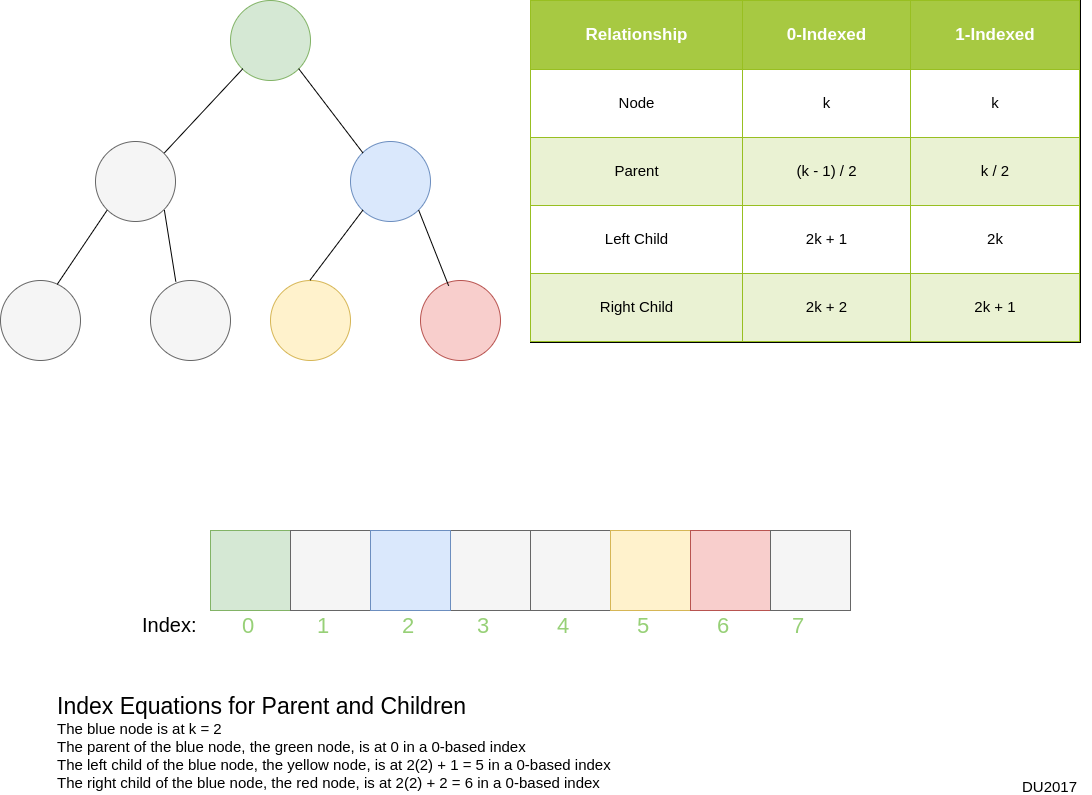Heapsort is one of my favorite sorting algorithms because it highlights the importance of considering the right data structures in algorithm design. Heapsort also has an upper-bound O(n log n) time complexity, which isn’t bad, since comparison-based sorting algorithms have a lower bound of Ω(n log n).

This post will explore the foundations of the heapsort – selection sort and the heap – before providing an overview of heapsort. Lastly, we will review both in-place and out-of-place implementations of heapsort in C# and Javascript.

## Selection Sort

Selection sort is an in-place, comparison-based sorting algorithm that builds the sorted array through incremental insertions, or swaps.

The in-place property of the algorithm is derived from dividing the targeted array into unsorted and sorted portions.

The algorithm works by linearly scanning the unsorted portion of the array for the largest item, subsequently swapping it with the last item in the unsorted section. This is followed by moving the partition, which reduces the range of the unsorted portion and increases the length of the sorted items.

This results in a worst-case and average-case quadratic time complexity, making it impractical on large arrays.

The O(n2) time complexity is caused by the consecutive linear scans for the largest item in the array. This is a direct effect from the data structure used in the algorithm: an array.

But there are other data structures that we can use to efficiently identify the largest item in a set, namely a binary heap.

## Binary Heap

A binary heap is a binary tree-based data structure that satisfies the heap property. In addition, since a binary heap is a type of heap, it is also an implementation of a priority queue.

First, the heap property states that for any node within the tree, it must dominate both of its children. In the case of a max-heap, any given node must have a greater value than both of its children. In the case of a min-heap, any given node must have a smaller value than both of its children.

As an implementation of a priority queue, an abstract data type, a binary heap supports the basic operations of Add, or Insert, in logarithmic time; FindMin, or FindMax, in constant time; and DeleteMin, or DeleteMax, in logarithmic time.## Array-Implemented Binary Heap

Binary heaps are most commonly implemented with an array as the underlying data structure, providing a space-efficient way to represent a binary tree without the space taken up by pointers.

Nodes within the binary tree are indexed in the array using the following equations:

Equation to find index of a node in a 1-based indexed array:
2l - 1 through 2l - 1
Where l is the level in the tree,
and the root level is at l = 1

Equation to find index of a node in a 0-based indexed array:
2l - 1 - 1 through 2l - 2
Where l is the level in the tree,
and the root level is at l = 1In addition, the index of the left and right child of a node, along with its parent, can be found using the following equations:

Formulas to find children and parent in 1-based indexed array:
k = the index of a node
left child = 2k
right child = 2k + 1
parent = k / 2

Formulas to find children and parent in 0-based indexed array:
k = the index of a node
left child = 2k + 1
right child = 2k + 2
parent = (k - 1) / 2### Limitations of a Binary Heap

The benefits gained from an array representation of a binary tree are in exchange for some limitations. It should be emphasized that an array implementation of a binary heap can only efficiently represent a binary tree, and not a binary search tree. The loss of pointers in an array implementation of a binary heap results in a loss of flexibility that affects its ability to efficiently reorganize subtrees.

Whereas in a binary search tree subtrees could be swiftly reorganized by pointing the root elsewhere, in an array this would require repositioning every individual element involved in the restructuring of the tree, making the ordeal cumbersome. And if we recall, an important condition to maintain the logarithmic look-up time in a binary search tree is its balance – and that requires performing rotations, which involves restructuring subtrees.

This leads us to the next limitation of an array implementation of a heap: there is no efficient search operation. Because a binary heap does not represent a binary search tree, there is a loose partial order on the items that results in the search operation having at least a linear lower bound time complexity.

But there are benefits to the loose partial order, which are seen in the aforementioned three basic operations of a priority queue: insert; find-min, or find-max; and delete-min, or delete-max.

## Heapsort Is Selection Sort with a Heap

Heapsort is basically the selection sort algorithm – an algorithm that builds a sorted array thorough incremental insertions – with the exception of using a heap. Recall that the usorted array in selection sort requires a linear scan to find the largest item to insert next in the sorted portion, resulting in a quadratic time complexity for the algorithm. A binary heap, on the other hand, identifies the largest item in constant time, but the follow-up replacemnt with the next largest item is done in logarithmic time, resulting in a O(n log n) time complexity, which is ideal for a comparison-based sorting algorithm.

The only downside to heapsort, which in my opinion isn't much of a downside, is that it requires more code to implement than a simple selection sort.This extra code is from having to build our own heap. So, let's check out some code for a heap class that I built in both C# and Javascript.

### C# Code

Here is the code for the Heap class:

``````public class Heap
{
private int[] Contents;
private int Size;
private int Items;

public Heap(int size)
{
this.Contents = new int[size];
this.Size = size;
this.Items = 0;
}

public Heap(int[] originalArray)
{
this.Heapify(originalArray);

this.Size = originalArray.Length;
this.Items = originalArray.Length;

}

public static void HeapifyArray(int[] arr, int lengthOfUnsorted, int position)
{
int dominant = position;
int leftChild = LeftChild(lengthOfUnsorted, position);
int rightChild = RightChild(lengthOfUnsorted, position);

if (leftChild != -1 && arr[leftChild] > arr[dominant])
{
dominant = leftChild;
}

if (rightChild != -1 && arr[rightChild] > arr[dominant])
{
dominant = rightChild;
}

if (dominant != position)
{
Swap(arr, position, dominant);
HeapifyArray(arr, lengthOfUnsorted, dominant);
}
}

private void Heapify(int [] a)
{
this.Contents = new int[a.Length];
for (int i = 0; i < a.Length; i++)
{
}

}

public bool IsEmpty()
{
return !(this.Items > 0);
}

public int TotalItems()
{
return this.Items;
}

{
if (this.Contents.Length == this.Items)
{
Console.WriteLine("The Heap is Full");
return;
}

Contents[this.TotalItems()] = value;
BubbleUp(this.TotalItems());
Items++;
}

public int ExtractMax()
{
int max = this.Contents;

this.Contents = this.Contents[this.Items - 1];
this.Contents[this.Items - 1] = 0;
this.Items--;
BubbleDown(0);

return max;
}

public int PeekMax()
{
return this.Contents;
}

private void BubbleUp(int position)
{
int parentPosition = Parent(position);
if (parentPosition == -1) { return; }

if (this.Contents[parentPosition] < this.Contents[position])
{
Swap(this.Contents, parentPosition, position);
BubbleUp(parentPosition);
}
}

private void BubbleDown(int position)
{
if(!IsLeaf(position))
{
if (
Contents[position] < Contents[LeftChild(this.Size, position)]
|| Contents[position] < Contents[RightChild(this.Size, position)]
)
{
if (Contents[RightChild(this.Size, position)] > Contents[LeftChild(this.Size, position)])
{
Swap(this.Contents, position, RightChild(this.Size, position));
BubbleDown(RightChild(this.Size, position));
}
else
{
Swap(this.Contents, position, LeftChild(this.Size, position));
BubbleDown(LeftChild(this.Size, position));
}
}
}
}

public static void Swap(int[] arr, int positionA, int positionB)
{
if (positionA == -1 || positionB == -1) { return; }

int contentsA = arr[positionA];
int contentsB = arr[positionB];
arr[positionA] = contentsB;
arr[positionB] = contentsA;
}

private int Parent(int position)
{
return position == 0 ? -1 : (position - 1) / 2;
}

public static int RightChild(int size, int position)
{
if (position == 0 && size > 2) { return 2; }
return (2 * position + 2) >= size ? -1 : (2 * position + 2);
}

public static int LeftChild(int size, int position)
{
if (position == 0 && size > 1) { return 1; }
return (2 * position + 1) >= size ? -1 : (2 * position + 1);
}

private Boolean IsLeaf(int position)
{
return (position >= ((this.TotalItems() / 2) + 1) && position <= this.TotalItems());
}

}
``````

Here is the code for the in-place and out-of-place heapsort methods:

``````public static int[] HeapsortOutOfPlace(int[] unsorted)
{
int[] sorted = new int[unsorted.Length];
Heap h = new Heap(unsorted);

for (int i = unsorted.Length - 1; i >= 0; i--)
{
sorted[i] = h.ExtractMax();
}

return sorted;
}

public static void Heapsort(int [] arr)
{
int lengthOfUnsorted = arr.Length;
int i = (lengthOfUnsorted / 2) - 1;

for (; i >= 0; i--)
{
Heap.HeapifyArray(arr, lengthOfUnsorted, i);
}

for (int t = lengthOfUnsorted - 1; t >= 0; t--)
{
Heap.Swap(arr, 0, t);
Heap.HeapifyArray(arr, t, 0);
}

}

``````

And here is the code that tests both heapsort methods:

``````public static void Main(string[] args)
{
Random random = new Random();

int[] unsortedArray = new int {5, 1, 2, 77, 12, 122, 98, 917, 1882, 42, 3, 19 };
Console.WriteLine(\$"Verifying unsorted order of initial array, expecting false: {VerifySortedOrder(unsortedArray)}");

int[] sortedArray = HeapsortOutOfPlace(unsortedArray);
Console.WriteLine(\$"Verifying sorted order of initial array with out-of-place heapsort, expecting true: {VerifySortedOrder(sortedArray)}");
Heapsort(unsortedArray);
Console.WriteLine(\$"Verifying sorted order of initial array with in-place heapsort, expecting true: {VerifySortedOrder(unsortedArray)}");

for (int t = 1; t < 4; t++)
{
int[] a = new int[t * 150];

for (int i = 0; i < 150 * t; i++)
{
a[i] = random.Next(1, 200000);
}

int[] sortedOutOfPlace = HeapsortOutOfPlace(a);
Console.WriteLine(\$"\nVerifying sorted order of array of size {t * 150} using out-of-place heapsort, expecting true: {VerifySortedOrder(sortedOutOfPlace)}");
Console.WriteLine(\$"Verifying unsorted order of array of size {t * 150}, expecting false: {VerifySortedOrder(a)}");
Heapsort(a);
Console.WriteLine(\$"Verifying sorted order of array of size {t * 150} using in-place heapsort, expecting true: {VerifySortedOrder(a)}");
}
}
``````

### Javascript Code

Here is the code the for the Heap class:

``````"use strict";

module.exports = class Heap {
constructor() {
this.Contents = [];
}

static heapifyArray(arr, lengthOfUnsorted, position) {
let dominant = position;
let leftChild = Heap._leftChild(position);
let rightChild = Heap._rightChild(position);

if (leftChild < lengthOfUnsorted && arr[leftChild] > arr[dominant]){
dominant = leftChild;
}

if (rightChild < lengthOfUnsorted && arr[rightChild] > arr[dominant]){
dominant = rightChild;
}

if (dominant != position) {
Heap._swap(arr, position, dominant);
Heap.heapifyArray(arr, lengthOfUnsorted, dominant);
}

}

heapify(arr) {
if (!arr || !Array.isArray(arr) || !arr.length) { return; }

this.Contents = [];

for (let i = 0; i < arr.length; i++) {
}
}

if (!value) { return; }

this.Contents.push(value);
this._bubbleUp(this.size() - 1);
}

extractMax() {
if (this.size() == 0) { return; }

let max = this.Contents;

this.Contents = this.Contents.pop();
this._bubbleDown(0);

return max;
}

peekMax() {
return this.Contents;
}

size() {
return this.Contents.length;
}

isEmpty() {
return this.Contents.length == 0;
}

_bubbleUp(position) {
let parentPosition = Heap._parent(position);

if (parentPosition == -1) { return; }

if(this.Contents[parentPosition] < this.Contents[position]) {
Heap._swap(this.Contents, parentPosition, position);
this._bubbleUp(parentPosition);
}
}

_bubbleDown(position) {
if(!this._isALeaf(position)) {
if (this.Contents[position] < this.Contents[Heap._leftChild(position)]
|| this.Contents[position] < this.Contents[Heap._rightChild(position)]) {
if (this.Contents[Heap._rightChild(position)] > this.Contents[Heap._leftChild(position)]) {
Heap._swap(this.Contents, position, Heap._rightChild(position));
this._bubbleDown(Heap._rightChild(position));
} else {
Heap._swap(this.Contents, position, Heap._leftChild(position));
this._bubbleDown(Heap._leftChild(position));
}
}
}
}

_isALeaf(position) {
return (Heap._leftChild(position) >= this.size() && Heap._rightChild(position) >= this.size());
}

static _parent(position) {
return position == 0 ? -1 : Math.floor((position - 1) / 2);
}

static _leftChild(position) {
return 2 * position + 1;
}

static _rightChild(position) {
return 2 * position + 2;
}

static _swap(arr, positionA, positionB) {
let contentsA = arr[positionA];
let contentsB = arr[positionB];
arr[positionA] = contentsB;
arr[positionB] = contentsA;
}

}
``````

Here is the code for in-place and out-of-place heapsort functions:

``````"use strict";

const Heap = require("./heap.js");

module.exports = {
heapsortOutOfPlace: function(unsortedArray) {
if (!unsortedArray || !Array.isArray(unsortedArray) || !unsortedArray.length) { return; }
let h = new Heap(),
sorted = [];

h.heapify(unsortedArray);

for (let i = unsortedArray.length - 1; i >= 0; i--) {
sorted[i] = h.extractMax();
}

return sorted;
},

heapsort: function(arr) {
if (!unsortedArray || !Array.isArray(unsortedArray) || !unsortedArray.length) { return; }
let lengthOfUnsorted = arr.length;
let i = Math.floor((lengthOfUnsorted / 2) - 1);

for (; i >= 0; i--) {
Heap.heapifyArray(arr, lengthOfUnsorted, i);
}

for(let i = lengthOfUnsorted - 1; i >= 0; i--) {
Heap._swap(arr, 0, i);
Heap.heapifyArray(arr, i, 0);
}
}
}
``````

And here is the code that tests both heapsort functions:

``````"use strict";

const Heap = require("./heap.js");
const sort = require("./heapsort");

const fs = require("fs");
const filePath = "../test.txt";

});

let unsortedFromFile = [];
let results;

rl.on("line", (line) => {
unsortedFromFile.push(parseInt(line));
});

rl.on("close", () => {
console.log("Testing verifySortedOrder with [1, 2, 5, 3, 6, 7, 8], expecting false: ", verifySortedOrder([1, 2, 5, 3, 6, 7, 8]));
console.log("Testing verifySortedOrder with [1, 2, 3, 5, 6, 7, 8], expecting true: ", verifySortedOrder([1, 2, 3, 5, 6, 7, 8]));

let sortedFromFile = sort.heapsortOutOfPlace(unsortedFromFile);
console.log("\nTesting sorted order from file: ", verifySortedOrder(sortedFromFile));
sort.heapsort(unsortedFromFile);
console.log("Testing in-place heapsort from file: ", verifySortedOrder(unsortedFromFile));

for (let t = 1; t < 4; t++) {
let a = [];
for (let i = 0; i < 150 * t; i++) {
a.push(randomNum());
}
let sortedA = sort.heapsortOutOfPlace(a);
console.log(`\nTesting sorted order with \${t * 150} random numbers: `, verifySortedOrder(sortedA));
console.log(`Verifying unsorted order of array with \${t * 150} random numbers, expecting false: `, verifySortedOrder(a));
sort.heapsort(a);
console.log(`Testing sorted order of in-place heapsort with \${t * 150} random numbers: `, verifySortedOrder(a));
}
});

function verifySortedOrder(arr) {
for (let i = 0; i < arr.length - 1; i++) {
if (arr[i] > arr[i + 1]) { return false; }
}

return true;
}

function randomNum() {
return Math.floor(Math.random() * (200000 - 1) + 1);
}

``````

## Thoughts on Code

While both versions of heapsort run in O(n log n) time, the regular in-place version does require less space. In fact, it should have O(1) space complexity, whereas the out-of-place version that I created should have a O(n2) space complexity.

Despite the drastic difference in the space complexity between the two versions, I still like the out-of-place verision for learning and understanding the algorithm, as the abstraction of the operations makes it easier to understand: first, a heap is created and then the extractMax method is continously called on the heap until the sorted array is incrementally built up. For those approaching this algorithm for the first time, this version gives you that high-level illustration of the algorithm.

There's also the benefit of the out-of-place version not mutating the original input, which could be of use in certain scenarios. But I'm certain revisions could be made to reduce the space complexity to O(n).

Still, in practice, the in-place heap-sort is probably your best choice because of the O(1) space complexity.

### Conclusion

Heapsort is a very practical sorting algorithm because it has an upper-bound time complexity of O(n log n), which if we recall, is the lower-bound time complexity of any comparison-based sorting algorithm. This means that heapsort belongs to the set of functions that perform as quick as any comparison-based sorting algorithm can perform.

In addition, this distinction of being a O(n log n) comparison-based sorting algorithm is derived from the choice of data structure in the algorithm. The logic behind heapsort is very similar to the logic in selection sort. But the key difference behind the upper-bound superlinear time in heapsort and the upper-bound quadratic time in selection sort is the tactic used to identify the largest item in the unsorted portion – which is a direct result from the choice of the underlying data structure used in the algorithm: it takes a linear scan to find the largest item in an unsorted array, but it takes logarithmic time to bubble up the largest item in a heap.

Besides being a very practical sorting algorithm, I appreciate heapsort because it is a reminder of the importance of choosing the right data structures in algorithm design, as it can make the difference between O(n2) and O(n log n) performance.